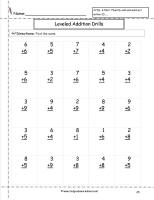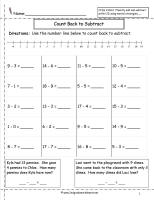﻿ CCSS 2.OA.2 Worksheets.  Addition and Subtraction Worksheets.

# Common Core State Standard 2.OA.2

## Operations & Algebraic Thinking

### Add and Subtract Within 20

2. Fluently add and subtract within 20 using mental strategies.  By end of Grade 2, know from memory all sums of two one-digit numbers.

###Single Digit Addition Worksheets

Common Core State Standards: 2.OA.2
Operations & Algebraic Thinking
Add and subtract within 20.

Fluently add and subtract within 20 using mental strategies. 2 By end of Grade 2, know from memory all sums of two one-digit numbers.

###Single Digit Addition Fluency Drills Worksheets

Common Core State Standards: 2.OA.2
Operations & Algebraic Thinking
Add and subtract within 20.

Fluently add and subtract within 20 using mental strategies. 2 By end of Grade 2, know from memory all sums of two one-digit numbers.

###Three Single Digit Numbers Addition Worksheets

Common Core State Standards: 2.OA.2
Operations & Algebraic Thinking
Add and subtract within 20.

Fluently add and subtract within 20 using mental strategies. 2 By end of Grade 2, know from memory all sums of two one-digit numbers.

###Single Digit Subtraction Worksheets

Common Core State Standards: 2.OA.2
Operations & Algebraic Thinking
Add and subtract within 20.

Fluently add and subtract within 20 using mental strategies. 2 By end of Grade 2, know from memory all sums of two one-digit numbers.